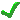## Course Software for Learning Differential Equations, Numerical Methods, and Linear Programming

### Coursewares

####How to solve problems of Mathematical Physics I

The courseware features chapters on algorithms of solutions of problems in Mathematical Physics:

• Ordinary Differential Equations
• Sturm-Lioville Eigenvalue Problems
• Partial Differential Equations
• Integral Transforms

####How to solve problems of Mathematical Physics II

The courseware features chapters on algorithms of solutions of problems in Mathematical Physics:

• Complex Analysis
• Probability and statistics
• Calculus of variations

####Scientific Computing with Matlab I

The courseware features four chapters on MATLAB numerical algorithms:

• Introduction into MATLAB computing
• Matrix computations and MATLAB linear algebra
• Numerical algorithms with MATLAB
• Mathematical modeling with MATLAB

####Scientific Computing with Matlab II

The computer labs feature twelve projects on scientific computing, including the project descriptions and MATLAB files.

### Software simulators

####Ordinary Differential Equations

The interactive software demonstrations feature three popular dynamical models:

• Nonlinear damped pendulum
• Predator-prey population system
• Van-der-Pol nonlinear oscillator

####Linear Programming and Network Flows

The interactive quizzes feature nine quizzes on problems of linear programming and network flows.

### Lecture notes

####Linear Algebra

The online lecture notes feature chapters on Linear Algebra:

• Linear systems of equations
• Matrices and determinants
• Eigenvalue problems
• Vector geometry
• Vector spaces

####Numerical Methods for Polynomial Interpolation

The online lecture notes feature three chapters on numerical methods and scientific computing:

• Introduction to numerical analysis and MATLAB programming
• Polynomial interpolation and approximation (curve fitting)
• Numerical differentiation and integration